alonsoformula In SpanishWhat are they?  |  How are they named? If we know the formula  | If we know the name  |  Examples  |  Exercises

What are they?

They are compounds formed by a metal and nonmetal.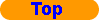How are they named?

For its nomenclature we use

Stock´s nomenclature: it is formed by two words. The first word is the name of the metal, followed immediately by the oxidation number between brackets and in Roman numbers. If the oxidation number of the metal is invariable, it is not indicated. The second word is the root of the nonmetal plus the suffix "–ide"

METAL(N) NONMETAL-ide

The IUPAC also accepts the stoichiometric nomenclature for these compounds, though it is better to use Stock´s nomenclature when there are metallic atoms and the stoichiometric nomenclature when all the atoms are nonmetals.If we know the formula

In the formula: The nonmetal has fixed oxidation number: halogens -1, chalcogens -2, group of N -3, group of C -4, and the oxidation number of the metal can be deduced by knowing that the compound is neutral. If it is always the same, we must know it and it is not necessary to deduce it.

How to determine the oxidation number of the metal?

1. Multiply the oxidation number of the nonmetal by its subscript. Change the sign of the result.
2. Divide this result by the subscript of the metal. This is the value of the oxidation number of the metal, and of the Roman number that you must use.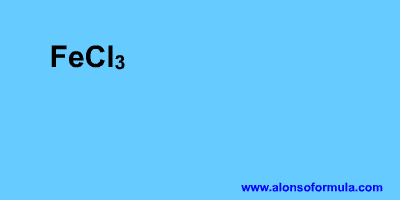If we know the name

In the name: Remember that you must know the symbols of the elements and the oxidation numbers that are invariable.

1. Write the symbol of the first element with the oxidation number; it is either between brackets or you have to know it.
2. Write the symbol of the second element with its oxidation number, which you have to know .
3. Calculate the fewest atoms of each element that you need so that the compound be neutral.Examples

 Stock´s nomenclature CaF2 Calcium fluoride CuBr2 Copper(II) bromide FeCl3 Iron(III) chloride K2Se Potassium selenide Ag2S Silver sulfideExercises

In Formulae you have an exercise to write the names of these substances and to check your results. You also have the answer to the exercise.

In Names you have an exercise to write the formulae for these substances and to check your results. You have to introduce the formulae without subscripts, for example for water = H2O. You also have the answer to the exercise.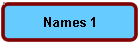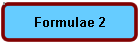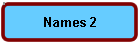Home | Introduction | Oxidation number | Mechanics | Kind of substances | Elementary substances | Metal oxides | Nonmetal oxides | Metal-nonmetal compounds | Nonmetal-nonmetal compounds | Metal hydrides | Hydracids | H with nonmetal | Hydroxides | Oxyacids | Oxysalts | Acid salts | Exercises | Periodic Table | Links
Author: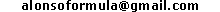WWW.ALONSOFORMULA.COM Formulación Inorgánica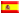Formulación OrgánicaFormulación Inorgánica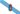Formulación OrgánicaFormulació InorgánicaFormulació OrgánicaEzorganikoaren Formulazioa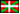Nomenclature of Inorganic Q.Física y Química de ESOFísica e Química de ESOFQ de 1º de BachilleratoFQ de 1º de BacharelatoQuímica de 2º de BachilleratoPrácticas de Química